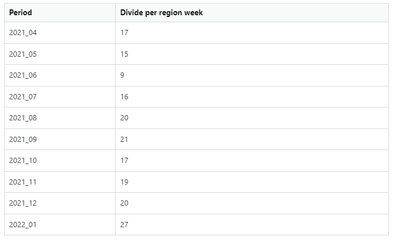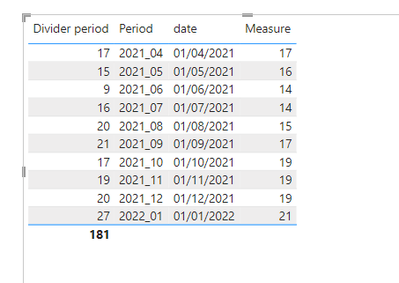cancel
Showing results for
Did you mean:Helper I

## Power BI Dax to Calculate the average in 3 periods

Hello,

I have two below tables in Dim_Date the Period doesn't correspond to exact months and first I calculated a measure to Divide the count of records per region by NumofWeeks.

The Num of the week is always the same in the same period.Divide per region week =
VAR TotalCountPerRegion = COUNT(Table1[Region])
VAR tblNumOfWeeksInPeriod=
SUMMARIZE(
DimDate
,DimDate[Period]
,DimDate[NumofWeeks]
)

VAR SuMOfWeeksInPeriod = SUMX(tblNumOfWeeksInPeriod,DimDate[NumofWeeks])

RETURN
MROUND(
DIVIDE(
TotalCountPerRegion
,SuMOfWeeksInPeriod
)
,1
)

With the formula, I get the following tableI want a new formula to calculate the average of the period with 2 following periods Example
Average 2021_04 = 2021_04 + 2021_05 + 2021_06 = (17+15+9)/3

Average 2021_05 = 2021_05 + 2021_06 + 2021_07 = (15+9+16)/3
and so on.

2 ACCEPTED SOLUTIONSHelper I

Thank you ...

I want to share a pbix file is it possible seems I don't have an option available to insert a file ...

am I missing some button?

My real measure is more complex because I already have a measure to apply to the 3-month average ...Helper I

I accepted this solution Thank you all

[M 3-Period Rolling Avg] =
// Set the number of periods to calculate
// the average over.
var PeriodCount = 3
// First, get the last visible period.
var LastPeriodSeqno = MAX( Dim_Date[PeriodSeqno] )
// Then, get the periods over which to calc.
var PeriodsToAverageOver =
CALCULATETABLE(
DISTINCT( Dim_Date[PeriodSeqno] ),
// Here's the place where you use the fact that
// all the periods are consecutively numbered.
// In fact, the counting does not have to start
// at 1 but it has to increment by 1.
Dim_Date[PeriodSeqno] <= LastPeriodSeqno,
Dim_Date[PeriodSeqno] > LastPeriodSeqno - PeriodCount,
REMOVEFILTERS( Dim_Date )
)
// We need to make sure that there are indeed
// PeriodCount periods in the set. Otherwise,
// the average will not be correct. This could happen
// if we were too close to the beginning of the calendar.
var ShouldCalculate =
COUNTROWS( PeriodsToAverageOver ) = PeriodCount
var Result =
if( ShouldCalculate,
CALCULATE(
AVERAGEX(
PeriodsToAverageOver,
[M]
),
REMOVEFILTERS( Dim_Date )
)
)
return
Result

7 REPLIES 7Community Support

Hi @ofeliajesus ,

Create a date column first.

``dat1e = DATE(LEFT(DimDate[Period],4),RIGHT(DimDate[Period],2),1)``

Then create a measure.

``````Measure11 =
VAR _MONTH =
( EDATE ( MAX ( DimDate[dat1e] ), -3 ) )
RETURN

AVERAGEX(FILTER((DimDate),DimDate[dat1e]>=_MONTH&&DimDate[dat1e]<=SELECTEDVALUE(DimDate[dat1e])),[Quotes per Period])``````

Best Regards
Community Support Team _ Polly

If this post helps, then please consider Accept it as the solution to help the other members find it more quickly.Helper I

In the Table Measures, I have a formula for Quotes Per period with the DAX that I need to use to apply in the rolling 3 periods starting in 2021_04
The Output will be
2021_06 - 2021_04 + 2021_05 + 2021_06 = (17+15+9)/3
2021_07 - 2021_05 + 2021_06 + 2021_07 = (15+9+16)/3
and so on

I started a new formula in the field trying to join the first calculation with the rolling 3 periods the formula is in Table Measures name Measure

thank youHelper I

I decided to copy/ paste it hereI have the following Dax

The divider period is a measure - I want to divide the count per region by number of weeks per period(

# of Weeks)

Divider Period =
VAR tblNumOfWeeksInPeriod=
SUMMARIZE(
Dim_Date
,Dim_Date[Period]
,Dim_Date[# of Weeks]
)

VAR SuMOfWeeksInPeriod = SUMX(tblNumOfWeeksInPeriod,Dim_Date[# of Weeks])

Var quotesperweek =
MROUND(
DIVIDE(
'Table Measures'[Total Region]
,SuMOfWeeksInPeriod
)
,1
)
Return
quotesperweek

Now I am trying to write the DAX to apply the average of the following 3 periods with the formula that I already had.ThanksCommunity Support

Hi @ofeliajesus ,

Best Regards
Community Support Team _ Polly

If this post helps, then please consider Accept it as the solution to help the other members find it more quickly.Community Support

Hi @ofeliajesus ,

I have create a simple sample. Please refer to it to see if it helps you.

Create a date column.

``date = DATE(LEFT('Table (2)'[Period],4),RIGHT('Table (2)'[Period],2),1)``

Then create a measure.

``````Measure =
VAR _MONTH =
( EDATE ( MAX ( 'Table (2)'[date] ), -3 ) )
RETURN
CALCULATE (
AVERAGE ( 'Table (2)'[Divider period] ),
FILTER (
ALL ( 'Table (2)' ),
'Table (2)'[date] >= _MONTH
&& 'Table (2)'[date] <= SELECTEDVALUE ( 'Table (2)'[date] )
)
)
``````If I have misunderstood your meaning, please provide your pbix file without privacy information and desired output.

Best Regards
Community Support Team _ Polly

If this post helps, then please consider Accept it as the solution to help the other members find it more quickly.Helper I

Thank you ...

I want to share a pbix file is it possible seems I don't have an option available to insert a file ...

am I missing some button?

My real measure is more complex because I already have a measure to apply to the 3-month average ...Helper I

I accepted this solution Thank you all

[M 3-Period Rolling Avg] =
// Set the number of periods to calculate
// the average over.
var PeriodCount = 3
// First, get the last visible period.
var LastPeriodSeqno = MAX( Dim_Date[PeriodSeqno] )
// Then, get the periods over which to calc.
var PeriodsToAverageOver =
CALCULATETABLE(
DISTINCT( Dim_Date[PeriodSeqno] ),
// Here's the place where you use the fact that
// all the periods are consecutively numbered.
// In fact, the counting does not have to start
// at 1 but it has to increment by 1.
Dim_Date[PeriodSeqno] <= LastPeriodSeqno,
Dim_Date[PeriodSeqno] > LastPeriodSeqno - PeriodCount,
REMOVEFILTERS( Dim_Date )
)
// We need to make sure that there are indeed
// PeriodCount periods in the set. Otherwise,
// the average will not be correct. This could happen
// if we were too close to the beginning of the calendar.
var ShouldCalculate =
COUNTROWS( PeriodsToAverageOver ) = PeriodCount
var Result =
if( ShouldCalculate,
CALCULATE(
AVERAGEX(
PeriodsToAverageOver,
[M]
),
REMOVEFILTERS( Dim_Date )
)
)
return
ResultAnnouncements#### Exclusive opportunity for Women!

Join us for a free, hands-on Microsoft workshop led by women trainers for women where you will learn how to build a Dashboard in a Day!#### Power Platform Conference-Power BI and Fabric Sessions

Join us Oct 1 - 6 in Las Vegas for the Microsoft Power Platform Conference.Top Solution Authors
Top Kudoed Authors
Users online (1,888)